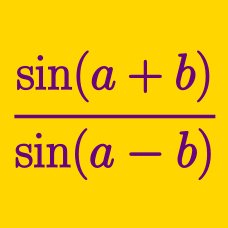Geometry

Sum and Difference Trigonometric Formulas: Level 4 Challenges

$\large \sin^{6}(\theta)+\cos^{6}(\theta)=\sin(2\theta) = \ ?$

If the above equation is true, then what is the value of $\sin(2\theta)$? Give your answer to 3 decimal places.$\Large\log_{\frac12}{\left(\displaystyle\prod_{n=1}^{45}\sin{(2n-1)}^{\circ}\right)}=\ ?$

$\tan \left [ \tan^{-1}\left(\frac12\right)+\tan^{-1}\left(\frac29\right)+\tan^{-1}\left(\frac18\right)+\tan^{-1}\left(\frac2{25}\right)+\tan^{-1}\left(\frac1{18}\right)+\ldots \right]$

What is the value of the expression above?

$\left(\sec^{2}10^{\circ}+\tan 10^{\circ}\right)\left(\sec^{2} 50^{\circ} - \tan 50^{\circ}\right)\left(\sec^{2} 70^{\circ}+\tan 70^{\circ}\right)$

The value of the expression above can be represented in the form $\dfrac{a-\sqrt{b}}{b}$ where $a$ and $b$ are positive coprime integers. Find the value of $a+b^2$.

$\large \displaystyle\prod_{r=1}^{44} (\cot (r^\circ) - 1)$

This product equals $2^{k}.$ Find $k.$

×